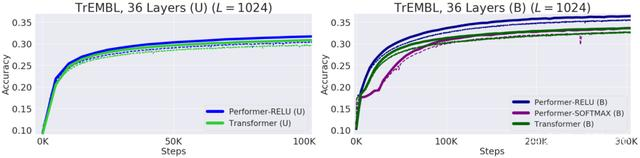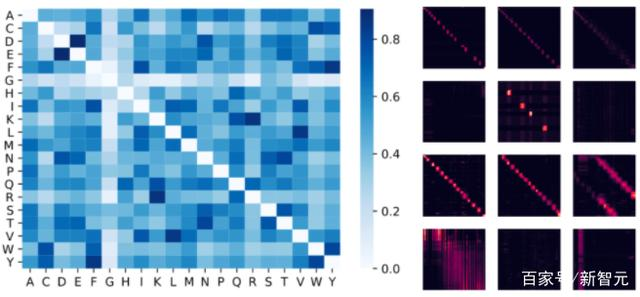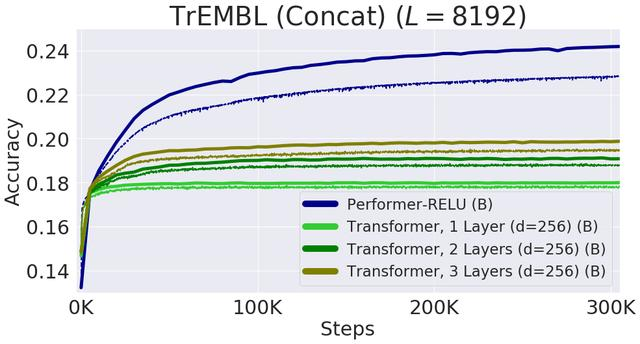# Performer¶

## 1. 介绍¶

### 1.1 基于Transformer模型应对长输入序列¶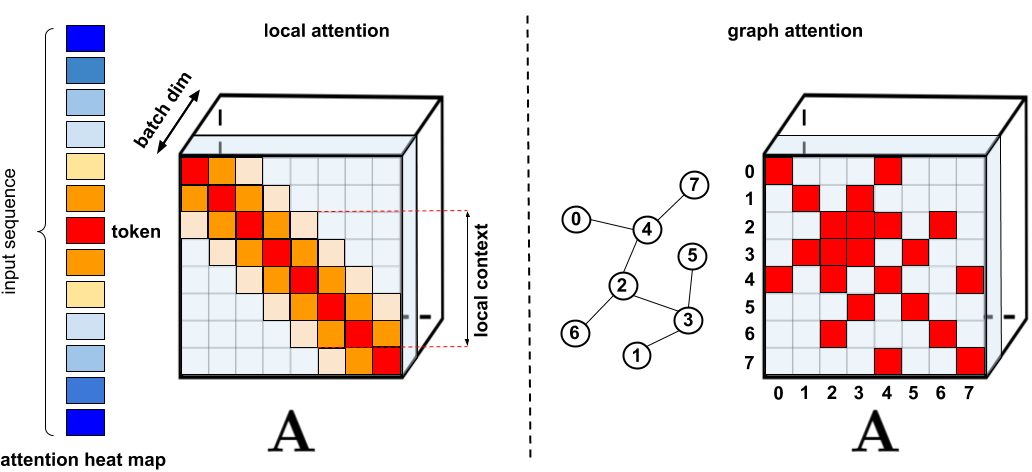• 需要高效的稀疏矩阵乘法运算，而这些运算并不是所有加速器都能提供。比如Longformer的实现采用TVM技术将计算自注意力的代码编译为CUDA核。

• 通常缺乏严格的理论保证。这点Big Bird是有的。

• 主要针对Transformer模型和生成式预训练进行优化。

• 通常需要堆叠更多的注意力层来补偿稀疏表征，这使得它们很难与其他预训练模型一起使用，因此需要进行再训练，这就很费时费力。

## 2. 两种常规的注意力机制回顾¶

$Att_{\longleftrightarrow}(Q,K,V)=D^{-1}AV$
$A=exp(\frac{QK^T}{\sqrt{d}})$
$D=diag(A1_{L})$

$Att_{\longleftrightarrow}(Q,K,V)=\tilde{D}^{-1}\tilde{A}V$
$\tilde{A}=tril(A)$
$\tilde{D}=diag(\tilde{A}1_{L})$

## 3. 广义注意力¶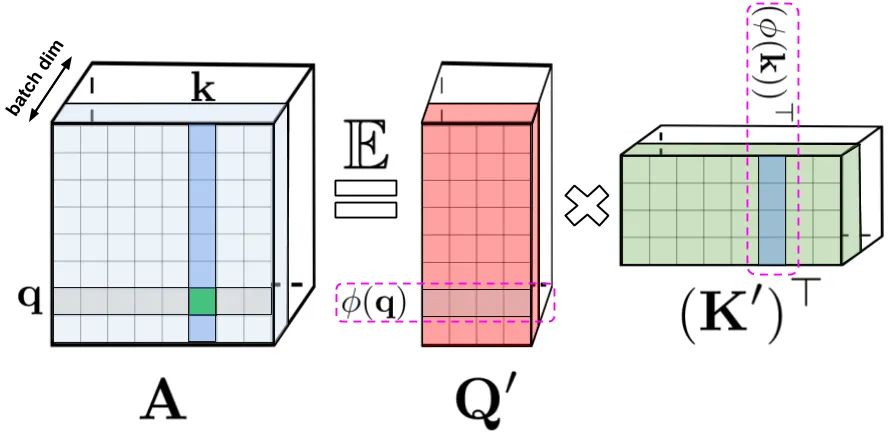LHS：标准注意力矩阵，由 query(表示为q) 和 key(表示为k) 上的softmax计算组成，表示两两之间的相似得分。RHS：标准注意力矩阵可以通过低阶随机化矩阵Q′和K′来近似，行编码由随机化非线性函数对原始query/key进行编码而得到。对于常规的softmax-attention，变换非常紧凑，涉及指数函数以及随机高斯投影。

## 4. FAVOR+：通过矩阵相关性实现快速注意力¶

### 4.1 FAVOR+理论¶

FAVOR+使用矩阵$$A \in \mathbb{R}^{L \times L}$$$$A(i,j)=K(q_{i}^T,k_{j}^T)$$，其中$$q_{i}/k_{j}$$代表的是Q/K中第$$i^{th}/j^{th}$$ 的query/key 行向量，核K：$$\mathbb{d}^d \times \mathbb{d}^d \rightarrow R_{+}$$ 定义为映射$$\phi$$:$$\mathbb{R}^d \rightarrow \mathbb{R}^r_{+}$$，公式为：

$K(x,y)=\mathbb{E}[(\phi(x)^T \phi(y))]$

$\widehat{Att\longleftrightarrow}(Q,K,V)=\widehat{D}^{-1}(Q^{'}((K^{'})^{T}V))$
$\widehat{D}=diag(Q^{'}((K^{'})1_{L}))$

$$\widehat{Att\longleftrightarrow}$$表示的是近似注意力，括号表示的是计算的顺序。很容易得到这个机制的空间复杂度为$$O(Lr+Ld+rd)$$，时间复杂度为$$O(Lrd)$$，而常规的注意力机制的空间复杂度为$$O(L^2+Ld)$$，时间复杂度为$$O(L^2d)$$

### 4.2 双向注意力近似¶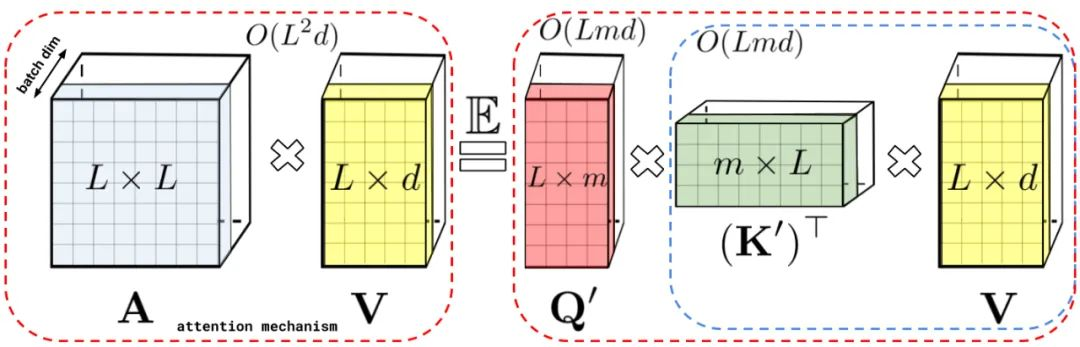### 4.3 单向注意力近似¶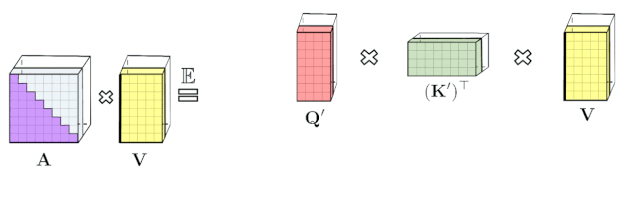### 4.4 softmax-kernels近似softmax¶

$$\phi$$用下面的形式表示，函数为$$f_{1},...f_{l}:\mathbb{R} \rightarrow \mathbb{R}$$， 函数$$g:\mathbb{R}^d \rightarrow \mathbb{R}$$

$\phi(x)=\frac{h(x)}{\sqrt(m)}(f_{1}(w_{1}^Tx),...,f_{1}(w_{m}^Tx),...,f_{l}(w_{m}^Tx))$

$h(x)=1,l=1, D=N(0,I_{d})$

$h(x)=1,l=2,f_{1}=sin,f_{2}=cos$

$D=N(0,I_{d})$

$SM(x,y)=exp(x^Ty)$

$SM(x,y)=exp(\frac{||y||^2}{2})K_{gauss}(x,y)exp(\frac{||y||^2}{2})$

$h(x)=exp(\frac{||x||^2}{2}),l=2,f_{1}=sin,f_{2}=cos$

## 5. 实验结果¶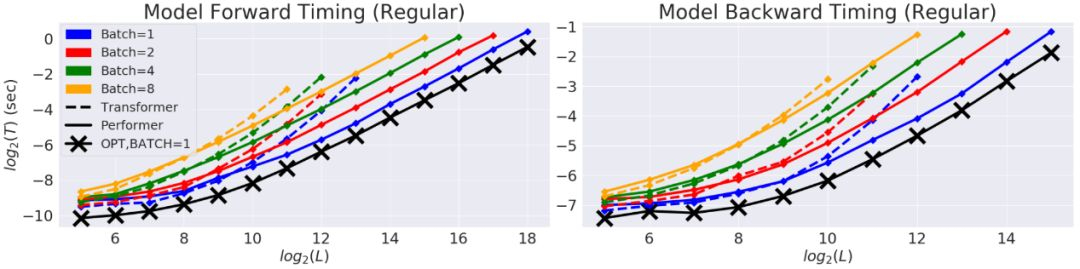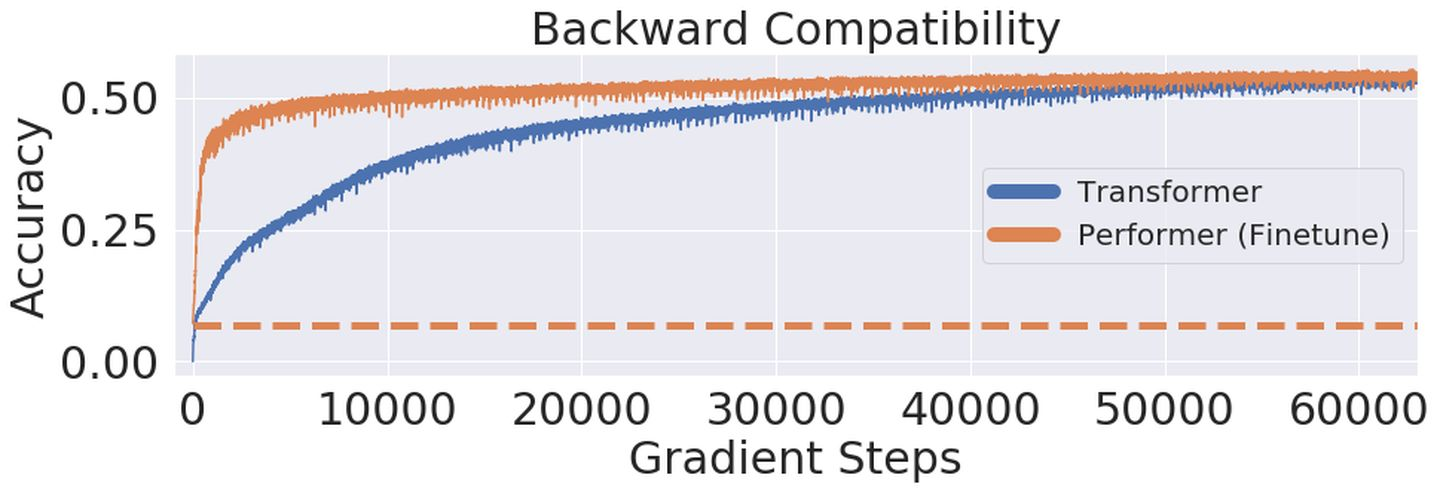## 6. 案例：蛋白质序列建模¶

Performer-ReLU (使用基于 relu 的注意力，这是一个不同于 softmax 的广义注意力)在蛋白质序列数据建模方面有很强的表现，而 Performer-Softmax 与 Transformer 的性能相匹配，正如理论所预测的结果那样。